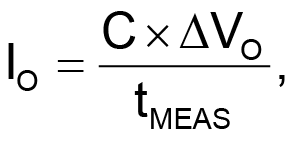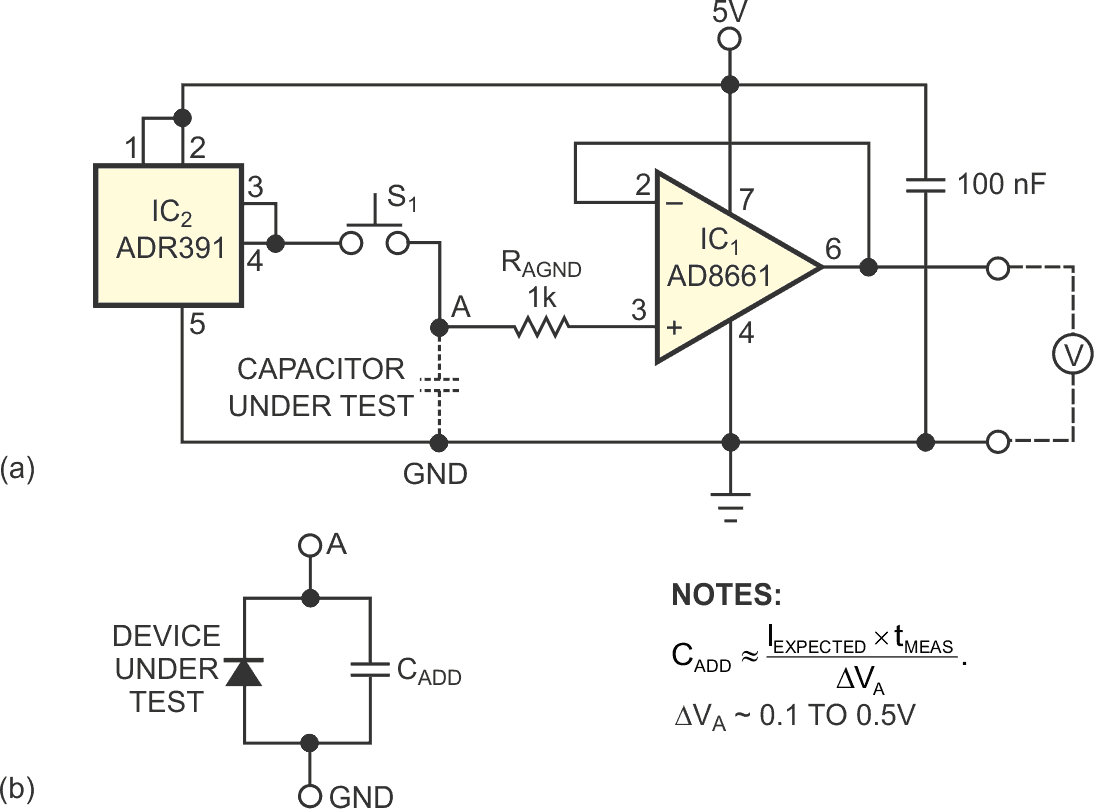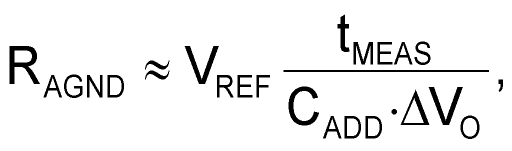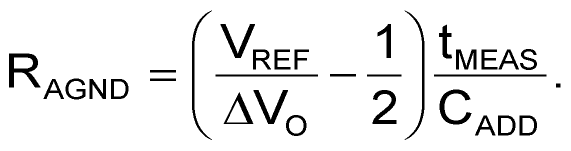# Simple Fixture Determines Leakage of Capacitors and Semiconductor Switches

Marian Stofka

EDN

The circuit in Figure 1a comprises a voltage follower, IC1, and the reference-voltage source of IC2. IC1 is an Analog Devices AD8661 op amp, which has a guaranteed input-bias current of no more than 1 pA and a typical input-bias current of 0.3 pA, and IC2 is an Analog Devices ADR391 precision voltage reference. The manufacturer trims the input offset voltage of this op amp not to exceed 100 µV, and the typical value is 30 µV. These properties suit this amplifier for observing self-discharging of almost any type of capacitor. The leakage currents of solid-tantalum capacitors and those having high-quality plastic dielectrics are well above the input-bias current of voltage follower IC1. The CUT (capacitor under test) initially charges to the reference-voltage level of 2.5 V by connecting Point A to the output of IC2. Subsequently, at some convenient time, Point A disconnects from the source of the reference voltage. A DVM (digital voltmeter) measures the output voltage of the follower at some reasonable time. The measured voltage drop, VO, with regard to initial value, should be 0.1 to 0.5 V. The leakage current,(1)

where C is the value of the CUT and tMEAS is the time between releasing the connection of the CUT to the 2.5 V source and the instant of readout at the voltage drop of VO.Figure 1. This simple fixture first impresses a reference voltage across a capacitor under test and then measures the voltage drop versus time at the output of the voltage follower (a). The circuit measures the leakage current of a reverse-biased active device (b).

The fixture also allows determining leakage currents of reverse-polarized diodes and of various switching devices in the off state, such as JFETs, MOSFETs, BJTs (bipolar-junction transistors), SCRs (silicon-controlled rectifiers), and IGBTs (insulated-gate bipolar transistors). In this case, the parallel combination of the DUT (device under test) and the added capacitor, CADD, replaces the CUT (Figure 1b). The measurement and the formula for evaluating the value of leakage current are the same as those for leakage current in the equation (1), but CADD substitutes for the CUT. A polystyrene-dielectric, 10-nF CADD works well for low-power devices. For high-power devices, however, the value of CADD should be at least 10 times the value of the parasitic capacitance of the DUT at 0 V.

Further, the fixture in Figure 1b can also determine the values of resistors of tens of megohms to about 2 TΩ. The current in the equation (1), in this case, is the current flowing through resistor RAGND at approximately the reference voltage. The resistance is roughly:or, more precisely:In all measurements, the voltage drop of VO should not exceed about one-fifth of the reference-voltage value to allow approximating the inherently exponential droop of VO by a linear decrease. The pushbutton switch in Figure 1a, S1, must exhibit a leakage of less than 1 pA. Stranded, isolated leads terminated with a gold-plated phosphorus-bronze pin can serve as a low-leakage switch. You can find gold-plated metal pieces in any type of high-quality connectors. Also, you can clip the DUT or CUT between two gold-plated clips made of similar connector parts. To minimize the circuit’s leakage, it uses no PCB.

EDN